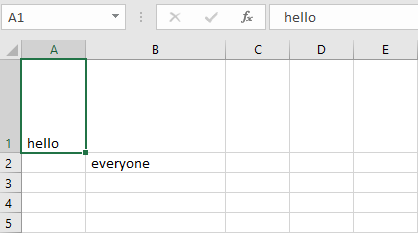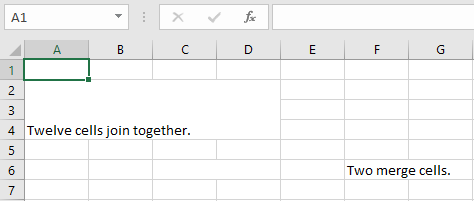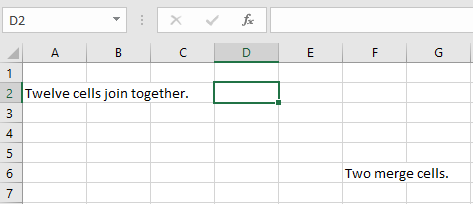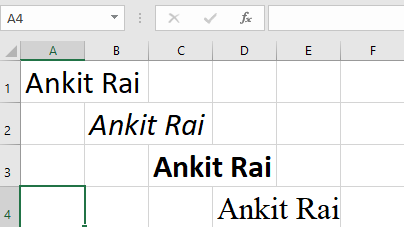Related Articles
Python | Adjusting rows and columns of an excel file using openpyxl module
• Difficulty Level : Medium
• Last Updated : 11 Jul, 2018

Prerequisites : Excel file using openpyxl writing | reading

#### Set the height and width of the cells:

Worksheet objects have `row_dimensions` and `column_dimensions` attributes that control row heights and column widths. A sheet’s `row_dimensions` and `column_dimensions `are dictionary-like values; row_dimensions contains RowDimension objects and column_dimensions contains ColumnDimension objects. In row_dimensions, one can access one of the objects using the number of the row (in this case, 1 or 2). In column_dimensions, one can access one of the objects using the letter of the column (in this case, A or B).

Code #1 : Program to set the dimensions of the cells.

 `# import openpyxl module``import` `openpyxl`` ` `# Call a Workbook() function of openpyxl ``# to create a new blank Workbook object``wb ``=` `openpyxl.Workbook()`` ` `# Get workbook active sheet  ``# from the active attribute. ``sheet ``=` `wb.active`` ` `# writing to the specified cell``sheet.cell(row ``=` `1``, column ``=` `1``).value ``=` `' hello '`` ` `sheet.cell(row ``=` `2``, column ``=` `2``).value ``=` `' everyone '`` ` `# set the height of the row``sheet.row_dimensions[``1``].height ``=` `70`` ` `# set the width of the column``sheet.column_dimensions[``'B'``].width ``=` `20`` ` `# save the file``wb.save(``'dimension.xlsx'``)`

Output:#### Merging the cells:

A rectangular area of cells can be merged into a single cell with the merge_cells() sheet method. The argument to `merge_cells()` is a single string of the top-left and bottom-right cells of the rectangular area to be merged.

Code #2 : Program to merge the cells.

 `import` `openpyxl``wb ``=` `openpyxl.Workbook()``sheet ``=` `wb.active`` ` `# merge cell from A2 to D4 i.e.``# A2, B2, C2, D2, A3, B3, C3, D3, A4, B4, C4 and D4 .``# A2:D4' merges 12 cells into a single cell. ``sheet.merge_cells(``'A2:D4'``)`` ` `sheet.cell(row ``=` `2``, column ``=` `1``).value ``=` `'Twelve cells join together.'`` ` `# merge cell C6 and D6``sheet.merge_cells(``'C6:D6'``)`` ` `sheet.cell(row ``=` `6``, column ``=` `6``).value ``=` `'Two merge cells.'`` ` `wb.save(``'merge.xlsx'``)`

Output:#### Unmerging the cells:

To unmerge cells, call the unmerge_cells() sheet method.

Code #3 : Program to unmerge the cells.

 `import` `openpyxl``wb ``=` `openpyxl.load_workbook(``'merge.xlsx'``)``sheet ``=` `wb.active`` ` `# unmerge the cells``sheet.unmerge_cells(``'A2:D4'``)`` ` `sheet.unmerge_cells(``'C6:D6'``)`` ` `wb.save(``'merge.xlsx'``)`

Output:#### Setting the font styles of the cells:

To customize font styles in cells, important, import the Font() function from the openpyxl.styles module.

Code #4 : Program to set the font of the text.

 `import` `openpyxl`` ` `# import Font function from openpyxl``from` `openpyxl.styles ``import` `Font`` ` `wb ``=` `openpyxl.Workbook()``sheet ``=` `wb.active`` ` `sheet.cell(row ``=` `1``, column ``=` `1``).value ``=` `"Ankit Rai"`` ` `# set the size of the cell to 24``sheet.cell(row ``=` `1``, column ``=` `1``).font ``=` `Font(size ``=` `24` `)`` ` `sheet.cell(row ``=` `2``, column ``=` `2``).value ``=` `"Ankit Rai"`` ` `# set the font style to italic``sheet.cell(row ``=` `2``, column ``=` `2``).font ``=` `Font(size ``=` `24``, italic ``=` `True``)`` ` `sheet.cell(row ``=` `3``, column ``=` `3``).value ``=` `"Ankit Rai"`` ` `# set the font style to bold``sheet.cell(row ``=` `3``, column ``=` `3``).font ``=` `Font(size ``=` `24``, bold ``=` `True``)`` ` `sheet.cell(row ``=` `4``, column ``=` `4``).value ``=` `"Ankit Rai"`` ` `# set the font name to 'Times New Roman'``sheet.cell(row ``=` `4``, column ``=` `4``).font ``=` `Font(size ``=` `24``, name ``=` `'Times New Roman'``)`` ` `wb.save(``'styles.xlsx'``)`

Output:Attention geek! Strengthen your foundations with the Python Programming Foundation Course and learn the basics.

To begin with, your interview preparations Enhance your Data Structures concepts with the Python DS Course.

My Personal Notes arrow_drop_up